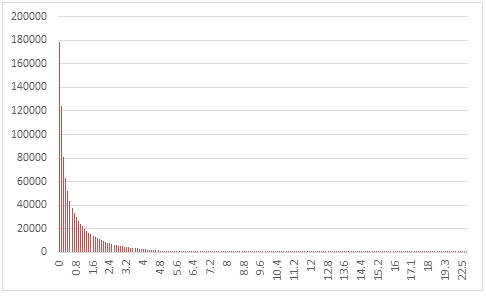# SQL Server random chi-squared distribution

RANDCHISQ

Updated: 31 March 2014

Use the table-valued function RANDCHISQ to generate a sequence of random numbers from a chi-squared distribution with @df degrees of freedom.
Syntax
SELECT * FROM [wctMath].[wct].[RANDCHISQ](
<@Rows, int,>
,<@df, float,>)
Arguments
@Rows
the number of rows to generate. @Rows must be of the type int or of a type that implicitly converts to int.
@df
the degrees of freedom. @df must be of the type float or of a type that implicitly converts to float.
Return Types
RETURNS TABLE (
[Seq] [int] NULL,
[X] [float] NULL
)
Remarks
·         @df must be greater than zero.
·         If @df is NULL then @df is set to 1.
·         If @MaxIterations is less than 1 then no rows are returned.
Examples
In this example we create a sequence 1,000,000 random numbers rounded to one decimal place from a chi-squared distribution with @df = 1, COUNT the results, paste them into Excel and graph them.
SELECT
X,
COUNT(*) as [COUNT]
FROM (
SELECT
ROUND(X,1) as X
FROM
wct.RANDCHISQ(
1000000, --@Rows
1     --@df
)
)n
GROUP BY
X
ORDER BY
X

This produces the following result.In this example we generate 1,000,000 random numbers from a chi-squared distribution with @df of 9. We calculate the mean, standard deviation, skewness, and excess kurtosis from the resultant table and compare those values to the expected values for the distribution.
DECLARE @size as int = 1000000
DECLARE @df as float = 9
DECLARE @mean as float = @df
DECLARE @var as float = 2*@df
DECLARE @stdev as float = SQRT(@var)
DECLARE @skew as float = SQRT(8e+00/@df)
DECLARE @kurt as float = 12e+00/@df

SELECT
stat,
[RANDCHISQ],
[EXPECTED]
FROM (
SELECT
x.*
FROM (
SELECT
AVG(x) as mean_CHISQ,
STDEVP(x) as stdev_CHISQ,
wct.SKEWNESS_P(x) as skew_CHISQ,
wct.KURTOSIS_P(x) as kurt_CHISQ
FROM
wct.RANDCHISQ(@size,@df)
)n
CROSS APPLY(
VALUES
('RANDCHISQ','avg', mean_CHISQ),
('RANDCHISQ','stdev', stdev_CHISQ),
('RANDCHISQ','skew', skew_CHISQ),
('RANDCHISQ','kurt', kurt_CHISQ),
('EXPECTED','avg',@mean),
('EXPECTED','stdev',@stdev),
('EXPECTED','skew',@skew),
('EXPECTED','kurt',@kurt)
)x(fn_name,stat,val_stat)
)d
PIVOT(sum(val_stat) FOR fn_name in([RANDCHISQ],[EXPECTED])) P

This produces the following result (your result will be different).

 stat RANDCHISQ EXPECTED avg 8.996961719 9 kurt 1.343216314 1.333333333 skew 0.944388767 0.942809042 stdev 4.23988631 4.242640687

### SupportCopyright 2008-2023 Westclintech LLC         Privacy Policy        Terms of Service